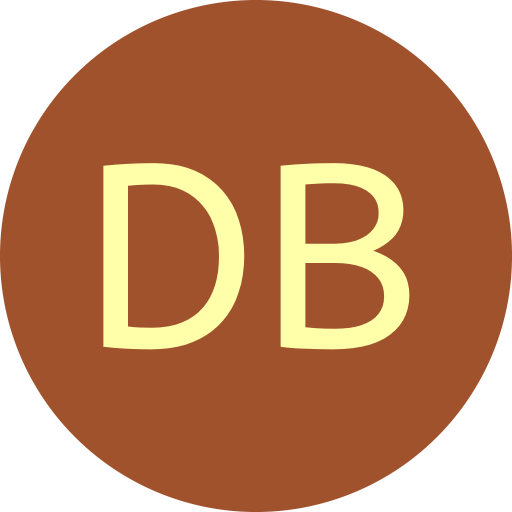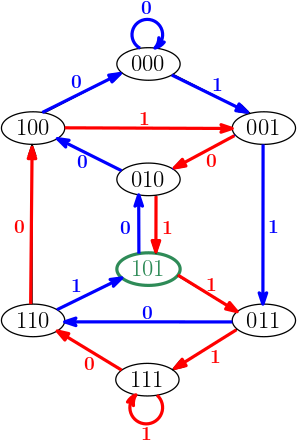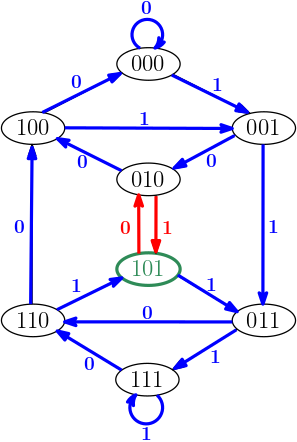De Bruijn Sequence and Universal Cycle Constructions

Recursive Construction Output
 Algorithm Recursive #1 Recursive #2 Order $n$ 1 2 3 4 5 6 7 8 9 10 11 12 13 14 15 16 17 18 19 20 21 22 23 24 25
Constructing DB sequences via recursion

DB sequences can be generated recursively by applying Lempel's D-morphism $D:\mathbf{B}(m) \rightarrow \mathbf{B}(m{-}1)$ which maps a binary string $\alpha = a_1a_2\cdots a_m$ to $\beta = b_1b_2\cdots b_{m-1}$ where each $$b_i = a_{i} \oplus a_{i+1}.$$ The mapping is onto, where both $\alpha$ and its complement $\overline{\alpha}$ map to the same $\beta$ [Lem70]. This mapping can be applied to cyclic (periodic) sequences in the obvious manner. When $\beta$ is a universal cycle (UC) for a set $\mathbf{S}$ consisting of $m$ binary strings with length $n$ then:

1. If $\beta$ has even weight, then $D^{-1}(\beta)$ consists of two disjoint UCs of order $n{+}1$ and length $m$.

2. If $\beta$ has odd weight, then $D^{-1}(\beta)$ consists of two rotations of the same UC of order $n{+}1$, length $2m$, and weight $m$.
For more details, see [Lem70,MW21]. Below are two methods that apply the above results to recursively construct DB sequences.

Recursive Construction #1

Let $\mathcal{D}$ be a DB sequence of order $n>1$. Since the weight (number of 1s) of $\mathcal{D}$ is even, let $D^{-1} = \{U, \overline{U} \}.$ The two UCs $U$ and $\overline{U}$ correspond to two edge-disjoint cycles in the underlying DB graph of order $n$ and they both contain the length-$n$ substring (vertex) $1010\cdots$. They can be joined together via this substring (vertex) to obtain a DB sequence of order $n{+}1$.

Example
 Given DB sequence $\mathcal{D} = 10111000$ of order $n=3$, then $$D(01101000) \ = \ D(10010111) \ = \ \mathcal{D}.$$ The DB graph of order 3 on the right highlights the two edge-disjoint cycles 01101000 and 10010111. The two cycles share the common vertex 101 (among others). After rotating the substrings $101$ to the front of each cycle, they can be joined together to obtain $$\mathbf{101}00001~\mathbf{101}11100,$$ which is a DB sequence of order $n{+}1=4$.Recursive Construction #2

Let $\mathcal{D}$ be a DB sequence of order $n>1$. Let $\mathcal{D}'$ denote the odd weight sequence obtained by replacing $1^n$ with $1^{n-1}$ in $\mathcal{D}$. Let $U$ denote the UC with length $2^{n+1}{-}2$ such that $D(U) = \mathcal{D}'$. Note that $U$ misses only the two alternating substrings $1010\cdots$ and $0101\cdots$ which appear in the cycle 01. The two cycles can be joined to obtain a DB sequence of order $n{+}1$.

Example
 Given DB sequence $\mathcal{D} =$ 10111000 of order $n=3$, then $\mathcal{D}' =$ 1011000 and $$D(01101111001000) = \mathcal{D}'.$$ The DB graph of order 3 on the right highlights the two edge-disjoint cycles 01101111001000 and 01. The two cycles share the common vertex 101. Joining these two cycles together yields the following DB sequence of order $n{+}1=4$: $$\mathbf{101}11100100001~\mathbf{10}.$$Like the Euler cycle and greedy approaches, an exponential amount of space is required by these recursive strategies. Optimized applications applying the D-morphism to construct DB sequences have been considered [Ann97,CPKS99]. A generalized recursive strategy for $k=2$ [Gam83] and a method for generalizing the D-morphism to larger alphabets $k>2$ [AA11] have also been studied.

References

• [AA11] A. Alhakim and M. Akinwande. A recursive construction of nonbinary de Bruijn sequences. Designs Codes and Cryptography, 60:155–169, 08 2011.

• [Ann97] F. Annexstein. Generating de Bruijn sequences: an efficient implementation. IEEE Transactions on Computers, 46(2):198–200, 1997.

• [CPKS99] T. Chang, B. Park, Y. H. Kim, and I. Song. An efficient implementation of the D-homomorphism for generation of de Bruijn sequences. IEEE Transactions on Information Theory, 45(4):1280–1283, 1999.

• [Gam83] R. Games. A generalized recursive construction for de Bruijn sequences. IEEE Trans- actions on Information Theory, 29(6):843–850, 1983.

• [MW21] C. Mitchell and P. R. Wild. Constructing orientable sequences, arXiv:2108.03069v1, 2021.

• [Lem70] A. Lempel. On a homomorphism of the de Bruijn graph and its applications to the design of feedback shift registers. IEEE Transactions on Computers, C-19(12):1204–1209, 1970.